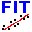#QtiSAS|QtiKWS

SANS: reduction, analysis, global instrumental fit

### Sidebar

#### JCNS :: Institutes

fit-curve# Nonlinear Fit.Curve(s) Interface

• Standard mode Simply fit example is shown below
• Fitting interface Fit.Curve(s) allows to fit a dataset taking into account polydispersity [of any parameter of a fitting function] and/or the instrument resolution in SA(N)S Fit mode.
• Global Fit algorithm is implemented, that allows simultaneously fit several curves with a mixute of global(common) and individual parametares.
• In Batch Fit (Set-by-Set) mode hundreds datasets could be fitted/re-fitted in “two mouse clicks”. This option is especially important for time resolved measurements, when datasets should be analyzed in the same way.
• Powerful Function Simulator simplifies the estimation of initial fitting parameters and the presentation of the obtained fitting curve(s).
• An advance of the fitting with QtiSAS is the runtime compilation of a fitting function in Fit.Compile . Program has possibility to check code of the function, change , and recompile it as a new function. The user-friendly interface Fit.Compile allows to create and compile a function of any complexity. GSL numerical algorithms could be easily integrated in the function body. The fitting function is written in C/C++, but option of calling of FORTRAN functions is implemented.

 “Simply Fit” Example: fit-curve-introduction.pdf cylinder-efit.fif data-cylinder.qti

/*

### The Chi-Square Minimization

Nonlinear curve fitting is an iterative procedure employing minimisation of the reduced chi-square value χ² to obtain the optimal parameter values. The reduced chi-square is obtained by dividing the residual sum of squares (RSS) by the degrees of freedom (DOF). Although this is the quantity that is minimized in the iteration process, this quantity is typically not a good measure to determine the goodness of fit. For example, if the y data is multiplied by a scaling factor, the reduced chi-square will be scaled as well.

M-Dimensional Data Sets [M>=1]

Residual Sum of Squares:

Reduced Chi-Square:

*/